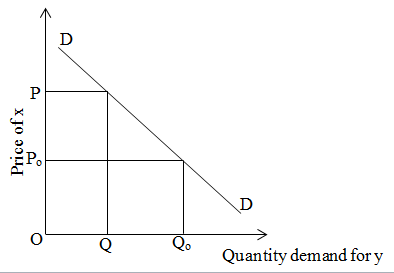# Negative cross elasticity of demand

In case of complementary goods cross elasticity of demand is negative because when the price of one commodity i.e x increases then demand for another commodity i.e. y decreases. We can explain it on the basis of given figure:On the above figure, in initial stage price of x is OP and quantity demand of y is OQ. When the price decreases from P to Po of x then demand for y increases from Q to Qo.